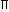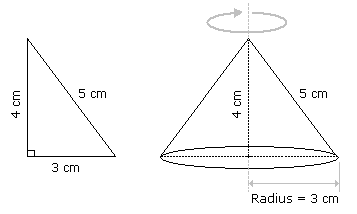# Aptitude - Volume and Surface Area - Discussion

Discussion Forum : Volume and Surface Area - General Questions (Q.No. 1)
1.
A right triangle with sides 3 cm, 4 cm and 5 cm is rotated the side of 3 cm to form a cone. The volume of the cone so formed is:
12cm3
15cm3
16cm3
20cm3
Explanation:Clearly, we have r = 3 cm and h = 4 cm.Volume = 1r2h =1 xx 32 x 4cm3 = 12cm3. 3 3

Video Explanation: https://youtu.be/Njsid2mVwUY

Discussion:
76 comments Page 1 of 8.

Stragi said:   1 year ago
As the base is 3.

How can the radius will be 3?

Why can't it (3) be a perimeter which is equal to 2*3.14*r.?

And also if it is rotated as base 3 then 4, 5 lengths will be slant heights and (4, 5 are not equal and can't make a perfect cone also).
(2)

Rakshitha A. R said:   2 years ago

Tamil said:   3 years ago
If we rotate 3 cm we can form a cone with height 4 cm and hypotenuses 5cm as per Pythagoras theorem and apply the volume of cone 1/3πr^2h.

Saurav said:   4 years ago
It is not mentioned that the 3cm is the base. The triangle is rotated about an axis that becomes height after the rotation then the answer will be 16pie.
(4)

Pavithra said:   4 years ago
If it is rotated along 3 then won't it be height?

How, h = 4? Explain me.

Shivam Ahuja said:   4 years ago
I think the answer may be 16π as per question, it does not tell us about along which axis we have to rotate it.

RAHUL robin said:   5 years ago
Incorrect/missing preposition in the question makes it confusing as one can't get whether the triangle is rotated with/about the side of 3 cm.

If it's rotated with the side of 3 cm then r=3, h=4. If it's rotated about the side of 3 cm then r=4, h=3.
(1)

Kaveri said:   5 years ago
It is;

r=3, h=4.
volume = 1/3 * 2 2/7 * r2 * h.
v = 1/3 * 22/7 * 9 * 4.
v = 12 * 22/7cm3.
(1)

Mohit kumar chaudhary said:   5 years ago